# LabVIEW Robotics Documents

cancel
Showing results for
Search instead for
Did you mean:

## An Introduction to A* Path Planning (using LabVIEW)

Introduction to the A* Algorithm

## Understanding the Example

Let’s start with a simple example to demonstrate the concept - suppose someone wants to move from point A to point B in the most efficient way possible. A wall separates the two points. In Figure 1, we see the field as we’ve described it.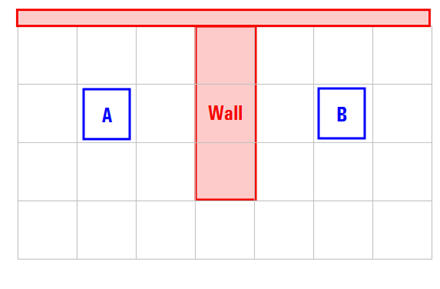Figure 1

We have divided our search area into a square grid. Simplifying the search area, as we have done here, is the first step in pathfinding. This particular method reduces our search area to a simple two dimensional array. Each item in the array represents one of the squares on the grid, and its status is recorded as walkable or unwalkable. The path is found by figuring out which squares we should take to get from A to B, and then walking from midpoint to midpoint (hereafter called the “nodes”) of each of the squares along the path. It is possible to divide up your pathfinding area into other shapes, but for this example, we will use squares since they are the simplest example.

## Starting the Search

Once we have simplified our search area into a manageable number of node, the next step is to conduct a search to find the shortest path. We do this by starting at point A, checking the adjacent node, evaluating the best next step, and continuing until we find B.

The A* implementation accomplishes this search by forming two lists.  The “open list” is a list of nodes that we are actively considering as potential candidates for our next step.  The closed list is a list of nodes that we have already evaluated and need not consider again.

To start, add the starting node to the closed list.

Look at all the nodes adjacent to the starting point, and add the walkable nodes to the open list while ignoring nodes that are not walkable.  In our case, all of the adjacent nodes are walkable, so we will add 8 new nodes to the open list. We also will assign a “parent” to each node (we’ll see why later) - here we’ll save point A as its "parent node".  In Figure 2, we see that the open list is outlined in green squares, and the parent node is noted by gray arrows.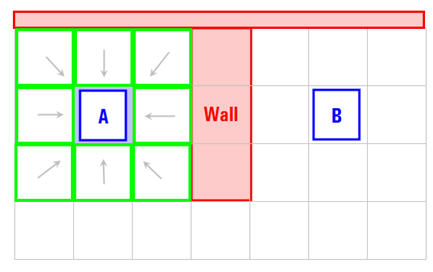Figure 2

## Path Scoring

The key to determining which nodes to use when figuring out the path is the following equation:

F = G + H

G = the movement cost to move from the starting point A to a given node on the grid

H = the estimated movement cost to move from that given node on the grid to the final destination, point B. This is often referred to as the heuristic

Our path is generated by repeatedly going through our open list and choosing the node with the lowest F score.

G is the movement cost to move from the starting point to the given node using the path generated to get there. In this example, we will assign a cost of 10 to each horizontal or vertical node moved, and a cost of 14 for a diagonal move. We use these numbers because the actual distance to move diagonally is the square root of 2 or roughly 1.414 times the cost of moving horizontally or vertically, so the ratio is correct and whole numbers are easier to manage (especially in a tutorial).

H can be estimated in a variety of ways. The method we use here is called the Manhattan method, where you calculate the total number of node s moved horizontally and vertically to reach the target node(B) from the current node, ignoring diagonal movement, and ignoring any obstacles that may be in the way. We then multiply the total by 10, our cost for moving one node horizontally or vertically.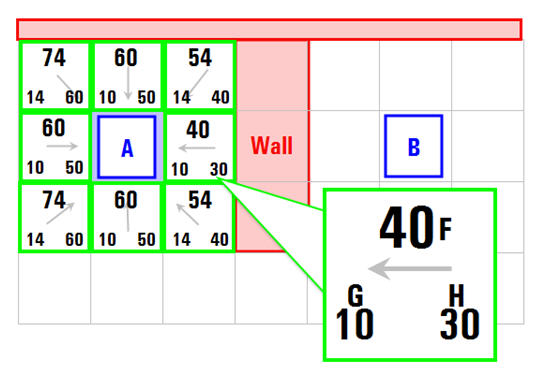Figure 3

## Finding the Path

In Figure 3, we can see the results of the scoring of our first round of nodes. In the node that we have highlighted, G = 10 because it is just one node from the starting node (A) in a horizontal direction. The nodes immediately above, below, and to the left of the starting node node all have the same G score of 10. The diagonal nodes have G scores of 14.

The H scores are calculated by estimating the Manhattan distance to the target node(B), moving only horizontally and vertically and ignoring the wall that is in the way. Using this method, the node to the immediate right of the start is 3 nodes from the target node (B), for a H score of 30. The node just above and to the right of the the starting node (A) is 4 nodes away (remember, only move horizontally and vertically) for an H score of 40.

Next, we choose the lowest F score node from all those that are on the open list move it to the closed list.  All of the adjacent nodes that are walkable should be added to the open list.  The nodes that are on the closed list should be ignored, and nodes that are already on the open list should be evaluated to see if this new path is a better one.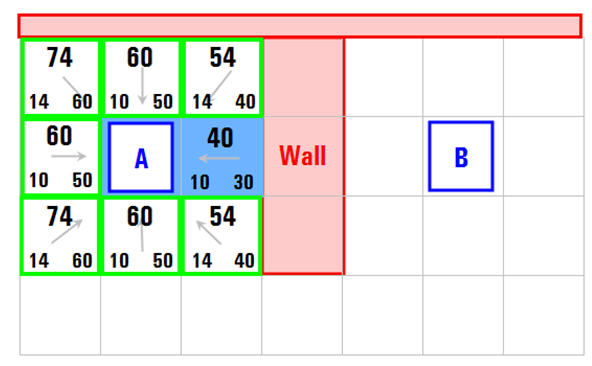Figure 4

In Figure 4, we can see this illustrated.  Of our initial 9 nodes, we have 8 left on the open list (outlined in green). Of these, the node with the lowest F cost is the one to the immediate right of the starting nodes, with an F score of 40.

We move it from our open list and add it to our closed list (that's why it's now highlighted in blue). Then we check all of its adjacent nodes. The ones to the immediate right of this square are inside the wall, so we ignore those. The one to the immediate left is the starting square. That's on the closed list, so we ignore that, too.

The other four nodes are already on the open list, so we need to check if the paths to those nodes are any better if you go through the "current" node to get there, using G scores as our point of reference. Let's look at the node right above the current node. Its current G score is 14. If we instead went through the current node to get there, the G score would be equal to 20 (10, which is the G score to get to the current node, plus 10 more to go vertically to the one just above it). A G score of 20 is higher than 14, so this is not a better path. That should make sense if you look at the diagram. It's more direct to get to that node from the starting node (A) by simply moving one node diagonally to get there, rather than moving horizontally one node , and then vertically one node.

When we repeat this process for all 4 of the adjacent nodes already on the open list, we find that none of the paths are improved by going through the current node, so we don't change anything. So now that we looked at all of the adjacent nodes, we are done with the current node, and ready to move to the next best node on the open list.

Repeat this process for all of the nodes on the open list, and you get the results shown in Figure 5.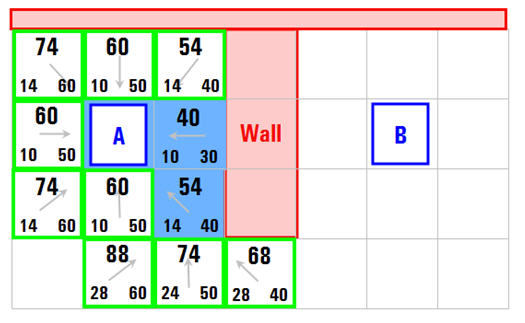Figure 5

To plan the complete path, you need to continue to repeat this process until you add the target node to the closed list, at which point the map would look like Figure 6.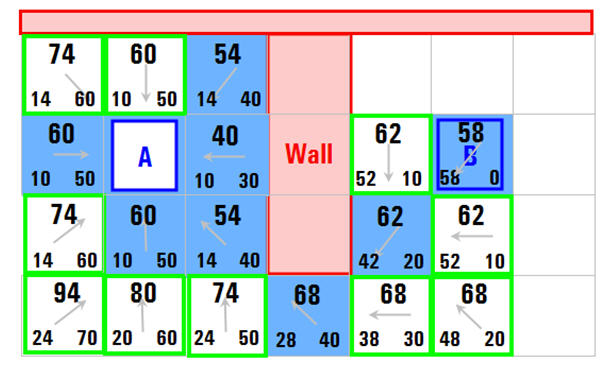Figure 6

Now to determine the path, just start at the target node, and work backwards moving from one node to its parent - in the diagram you can see this by following the arrows. This will eventually take you back to the starting node. It should look like the Figure 7.  This path is the least cost path from the starting node (A) to the target node (B).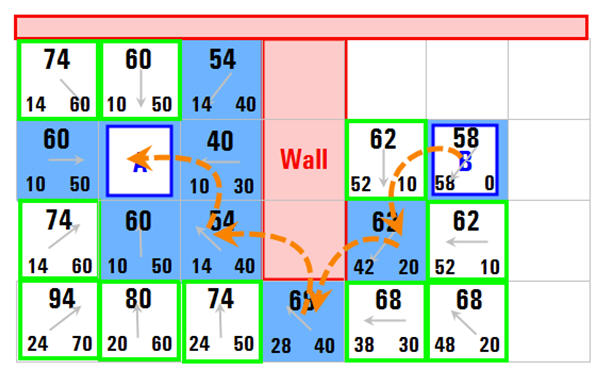Figure 7

# Implementing A* in LabVIEW

The LabVIEW Robotics Module includes various path planning algorithms including A*, so the remainer of this tutorial will reference an example – building a simple maze and finding the best path to the end of the maze.  In Figure 8, we see the end result.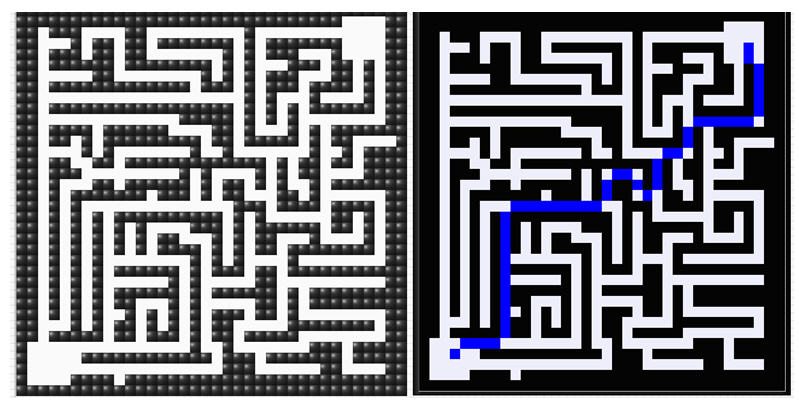Figure 8

## Building the Map

In LabVIEW, a map is represented as a 2D array of non-zero values.  Each value corresponds with a cost associated with moving through that node.  This allows you to not only represent “walkable” and “unwalkable” areas, but also assign values to the walkable areas to signify how difficult they are to walk.  For example, imagine that we’re developing a mapping algorithm akin to Google Maps. By assigning lower cost to highways than you do side streets, you can influence the results of the algorithm so that the path includes highways when possible and side streets only when necessary.  If this were not possible, the algorithm would always provide the shortest absolute pate regardless of traffic patterns.

In our LabVIEW example, we are providing a simple map of two values, so we are using a 2D array of Boolean controls to serve as the map input.  Thus, the possible costs are either 100 or 1.  This portion of the code is shown in Figure 9.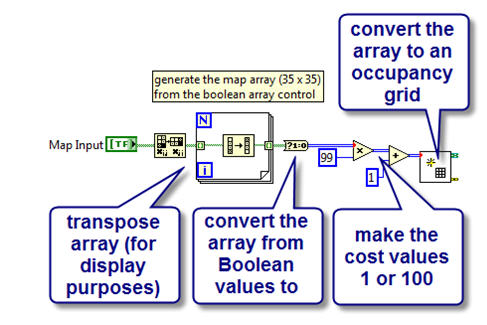Figure 9

Once the map is created, we can plan the path.  To do so, we just need to know the starting and ending locations on  the map, and the A Star Plan VI will provide the best path according to the A* algorithm.  In Figure 10, we see the path planning portion of the LabVIEW code.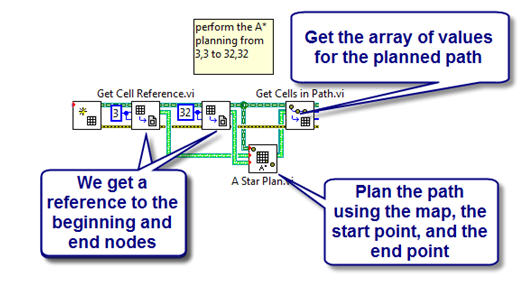Figure 10

Finally, we do some simple array manipulation for display, and we use an intensity map to show the maze and the planned path.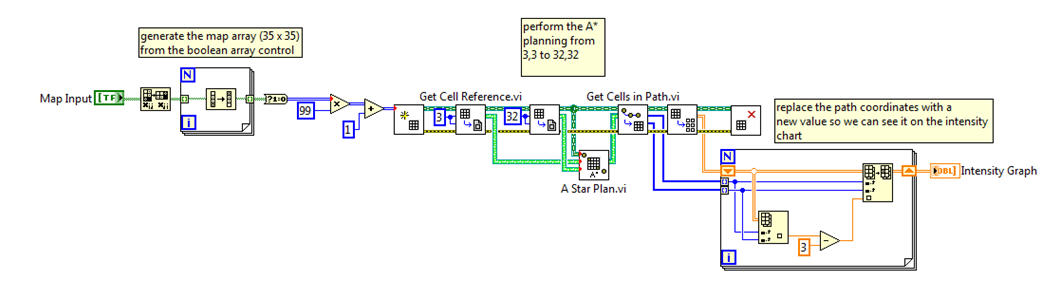Figure 11

The final code is attached, and it requires the LabVIEW 2009 Robotics Bundle.  For more information on sensors, drivers, decision making, or motor control with LabVIEW, visit ni.com/robotics.

CommentsMember
on

I don't know if it is it just me or is the following  true for all:

"...The H scores are calculated by estimating the Manhattan distance to the red target square..."

is the target square's colour meant to be "blue" instead of "red?"

Relative reference terms like "current node", "this node', "that node" managed to confuse me. Instead, if you had used absolute reference terms like node (1,2) [an instance of node (x,y)] would have been less ambiguous even though the reading "cost" would have been higher!! It would have made the already excellent presentation a little better!! Thank you.NI Employee (retired)
on

Thanks for the feedback!  I fixed that typo that you mentioned and tried to clean up some of the non specific pronouns (I can see how it was a little confusing).Member
on

Hello;

I am just learning the A* algorithm. But I think, (Assuming that left bottom corner node is (1,1)), The node (2,4) must be in the closed list in Fig.6 and Fig.7. Beacuse its cost is 60 and it had to be checked before (4,1) and (5,2) beacuse their costs are  68 and 62 respectively, which are higher than 60. Am I right?NI Employee (retired)
on

Wow, nice description of a fairly complex AI topic PJ!

Jervin Justin
NI TestStand Product ManagerNI Employee (retired)
on

Good call!  I'll see if I can update the diagram.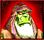Active Participant
on

thanks ,.....help . i can make  for the implementing in a  robot "starte KIT" robotics

Ing. Jonathan E. Cruz Ortiz

Cel : (+57) 3173669343 - (+57) 3124451894Member
on

this is very helpful. however, how can i use the path generated by the program to guide the robot ?

can you please reply this. thank youMember
on

The easiest way is to use the coordinates of the path as moving goals in the VFH algorithm (included with LabVIEW Robotics).  That way you use the map to create the desired path, and then VFH to follow it, which allows you to avoid any obstacles you didn't detect on your map.

There are some other more advanced approaches that consider the arc of the robot takes as well as replanning.  Here are some references to get you started if you want to look into using something more advanced:

http://scholar.lib.vt.edu/theses/available/etd-01162006-112326/unrestricted/AnkurThesis.pdf

http://www.recentonline.ro/028/Petrov_R28.pdf

http://www.flux.utah.edu/~flikx/publications/Flickinger_Daniel_thesis_20071012.pdf

http://www.cs.cmu.edu/~motionplanning/papers/sbp_papers/s/stentz_D2.pdfMember
on

How can i implement A* algorithm in starterkit 2.0.??Member
on

Can this algorithm be implemented on starter kit 2.0?Member
on

Is necessary more than 1 archive? Doesn't workMember
on

Hello everybody, an example of A * Algorithm implemented on Starter kit 2.0, DaNI, pleaseMember
on

UP :

How can i implement A* algorithm in starterkit 2.0.??Member
on

Is it possible to use for online path planning algorithmsMember
on

Como Puedo APLICAR Un algoritmo  *  en starterkit 2.0Member
on

Learning, thank you!Member
on

goodMember
on

Marker, thanks!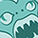Member
on

What should I do if the route needs to keep a certain distance from obstacles?

as the picture showsContributors